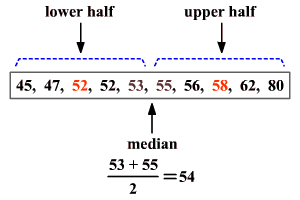# Interquartile, Semi-Interquartile and Mid-quartile Ranges

In a set of data, the quartiles are the values that divide the data into four equal parts. The median of a set of data separates the set in half.The median of the lower half of a set of data is the lower quartile ( $\text{L}\text{Q}$ ) or ${Q}_{1}$ .

The median of the upper half of a set of data is the upper quartile ( $\text{U}\text{Q}$ ) or ${Q}_{3}$ .

The upper and lower quartiles can be used to find another measure of variation call the interquartile range .

The interquartile range or $\text{IQR}$ is the range of the middle half of a set of data. It is the difference between the upper quartile and the lower quartile.

Interquartile range = ${Q}_{3}-{Q}_{1}$

In the above example, the lower quartile is $52$ and the upper quartile is $58$ .

The interquartile range is $58-52$ or $6$ .

Data that is more than $1.5$ times the value of the interquartile range beyond the quartiles are called outliers .

Statisticians sometimes also use the terms semi-interquartile range and mid-quartile range .

The semi-interquartile range is one-half the difference between the first and third quartiles. It is half the distance needed to cover half the scores.  The semi-interquartile range is affected very little by extreme scores.  This makes it a good measure of spread for skewed distributions. It is obtained by evaluating $\frac{{Q}_{3}-{Q}_{1}}{2}$ .

The mid-quartile range is the numerical value midway between the first and third quartile.  It is one-half the sum of the first and third quartiles.  It is obtained by evaluating $\frac{{Q}_{3}+{Q}_{1}}{2}$ .

(The median, midrange and mid-quartile are not always the same value, although they may be.)